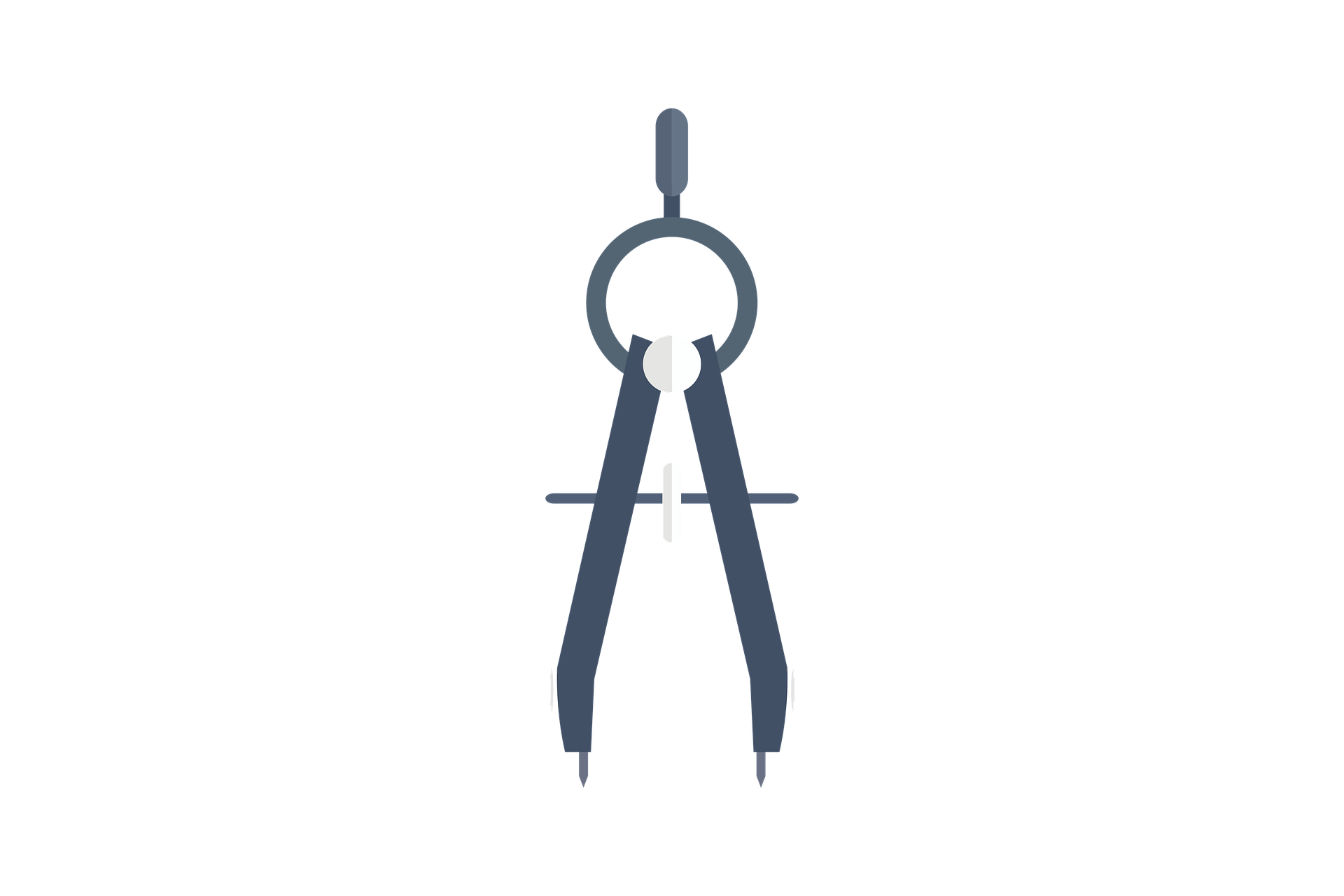# Geometry\$60.00
\$100.00

Dex Math provides a complete online Geometry course. Perfect for students who need to catch up on their Geometry skills, we also offer a personalized tutoring and homework assistance for your kids needs.

Geometry is the fourth math course in high school and will guide you through among other things points, lines, planes, angles, parallel lines, triangles, similarity, trigonometry, quadrilaterals, transformations, circles and area.

This Geometry math course is divided into 10 chapters and each chapter is divided into several lessons. Under each lesson you will find theory, examples and video lessons

What You'll Learn

• Points, Lines, Planes and Angles
• Proof
• Perpendicular and parallel
• Triangles
• Similarity
• Right triangles and trigonometry